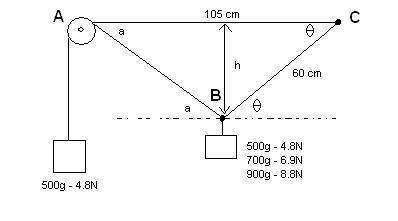# Static Forces. Angle problem.

1. The problem statement, all variables and given/known data

I drew up a little diagram to illustrate the problem.The goal of this lab report is to find the h value (in cm) for each of the possible masses at B. (one problem for 500g, one for 700g and one for 900g. The only thing that should change is the h value. The leftmost mass never changes)
θ and a are unknown. The pulley on the left is considered to be frictionless and the cords masses are negligible.

Point B is "tied" so the cord does not slide and thus BC is fixed at 60 cm. Length AB however is variable due to the pulley.

2. Relevant equations

Sin(θ) = opposite/hypotenuses

3. The attempt at a solution

Here are the equations I've come up with thus far.

Cos(a) = ABx/4.9N
Sin(a) = ABy/4.9N
ABx/Cos(a) = ABy/ Sin(a)

cos(θ) = (105-d)/60 (d being the top side of the left-most triangle, separated at h)
tan(a) = h/d
h = sqrt( (60^2) - ((105-d)^2) )

I can also say that:
BCx = ABx (as this is a static question. B is at rest, thus all resulting forces acting on it are equal to 0)
BCy + ABy - W = 0
or alternatively
BCy + ABy = W
(where W is the current mass of the object at point B)

I "know" the solution lies in the angles, but for the life of me I just can't fit my equations together in order to draw a definite conclusion. I'd love a push in the right direction if anyone can contribute something to this. I keep trying to break down the forces but without an angle it is difficult and I can't find the proper substitution.

First time post here, I hope I did it right, thanks in advance for any help.

#### KLoux

I "know" the solution lies in the angles, but for the life of me I just can't fit my equations together in order to draw a definite conclusion. I'd love a push in the right direction if anyone can contribute something to this. I keep trying to break down the forces but without an angle it is difficult and I can't find the proper substitution.
It seems that you're on the right track...

Take a step back before trying to solve for the angle. What are the forces acting on the two blocks? How do these forces relate to the angles?

-Kerry

Last edited:

It has been a week and I still seem to be unable to get the answer myself.
A collegue did the calculations and passed them to me, but they seem to essentially be my own calculations and don't give an h value (which we are searching for)
I plugged them into Maple 12 and it cannot solve it. I am quite certain I have too many unknowns and I cannot figure out how to elemenate them.

If someone could at least confirm I am not missing any formulas or maybe which angle to focus on? (I would figure I need to 'link' the formula's using the angles, but have yet to figure out how)

Thanks again.

Oh, and the formula's he gave me are...

-4.905*cos(a) - Tr cos(q)=0

-4.905 + 4.905*sin(a)+Tr*sin(q)=0

.6/(sin(a))= 1.05/(sin(180 - (a +q))

Last edited:

#### tiny-tim

Homework Helper
Welcome to PF!

Hi Madcat! Welcome to PF!You know T, and you know that tan(a) = h/(105 - 60cosθ).

You don't know the tension in the 60 string, and you don't want to know it, so … standard trick … take components perpendicular to that string.

What do you get?### The Physics Forums Way

We Value Quality
• Topics based on mainstream science
• Proper English grammar and spelling
We Value Civility
• Positive and compassionate attitudes
• Patience while debating
We Value Productivity
• Disciplined to remain on-topic
• Recognition of own weaknesses
• Solo and co-op problem solving